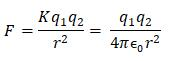Courses

# Test: Electric Field Intensity And Electric Field Calculations

## 20 Questions MCQ Test Physics Class 12 | Test: Electric Field Intensity And Electric Field Calculations

Description
This mock test of Test: Electric Field Intensity And Electric Field Calculations for Class 12 helps you for every Class 12 entrance exam. This contains 20 Multiple Choice Questions for Class 12 Test: Electric Field Intensity And Electric Field Calculations (mcq) to study with solutions a complete question bank. The solved questions answers in this Test: Electric Field Intensity And Electric Field Calculations quiz give you a good mix of easy questions and tough questions. Class 12 students definitely take this Test: Electric Field Intensity And Electric Field Calculations exercise for a better result in the exam. You can find other Test: Electric Field Intensity And Electric Field Calculations extra questions, long questions & short questions for Class 12 on EduRev as well by searching above.
QUESTION: 1

### Two point charges 2μC and 8μC are placed 12 cm apart. The position of point from 2μC charge, where the electric field intensity is zero is:

Solution:

Let net electric is zero at point P at a distance r from the 2mc charge.
Electric field at point P due to charge 2mc i sin direction of 8mc and its value is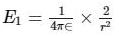Electric field at point P due to charge 8mc isin direction of 2mc and its value is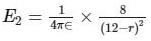for net field to be zero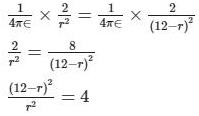taking root on both sides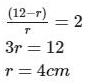QUESTION: 2

### Two equal positive charges q1 = q2 = 2.0 μC. are located at x = 0, y = 0.3 and x = 0 and y = -0.3 m respectively. What are the magnitude and direction of the total electric force (expressed in Newton and degrees counter clockwise w.r.t  x - axis) that q1 and q2 exert on a third charge Q = 4.0 μC  at x =0.4 and y = 0 m

Solution: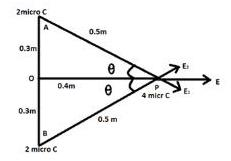Side AP =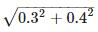= 0.5m Electric Field due to charge at A on point P = Elecric Field due to charge at B on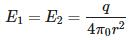point P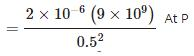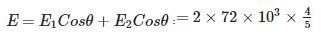Where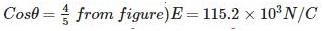So force due to this field on charge P

F = qE =  4 x 10-6 x 115.2 x 103 F = 0.46N

Since this force in the direction of positive x axis so angle  = 0o

QUESTION: 3

### The electric field and force experienced by a test charge placed at origin is 6 x 105N/C and 18 x 10-4 N. The value of test charge is:​

Solution:

By definition of Electric Field,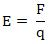QUESTION: 4

A tennis ball which has been covered with charges is suspended by a thread so that it hangs between two metal plates. One plate is earthed, while other is attracted to a high voltage generator. The ball

Solution:

The plate which connected to high voltage generator induces negative charge on ball which causes attraction. When the ball strikes the positive plate, charge distribution again takes place that is the bass becomes positive and repulsion takes place. When it strikes the plate which connected to earth than its charge goes to earth and again it will be attracted towards positive plate. Hence the ball swings backward and forward hitting each plate in turn.

QUESTION: 5

Which, among the following is the correct expression for electric field?

Solution:

Electric field is the force per unit charge hence, the correct expression is: E=F/Q.

QUESTION: 6

A charge q is placed at the center of the line joining two equal charges Q. The system of the three charges will be in equilibrium if q is equal to

Solution: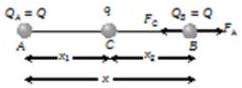Suppose in the following figure, equilibrium of charge B is considered.

Hence for its equilibrium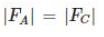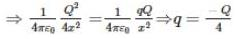QUESTION: 7

Electric field intensity varies with distance as:

Solution:

E = F/q = KQ/r2

QUESTION: 8

If electric field lines cross each other that would mean

Solution:

Properties of Electric Field Lines. At every point on an electric field line, the tangent represents the direction of electric field. If two field lines cross each other at the point, then there would be two possible directions for electric field which is physically impossible.

QUESTION: 9

The S.I. unit of electric field intensity is:​

Solution:

Electric Field Intensity E = F\q

QUESTION: 10

The diagram shows the electric field lines in a region of space containing two small charged spheres, Y and Z then which statement is true?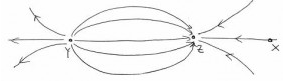Solution:

Electric Field Lines originate from positive charge and terminate at negative charge. The relative number of field lines originating from each charge represents the magnitude of the charge.

QUESTION: 11

Which equation correctly shows the force on a charge q in an electric field E

Solution:

E = F/q

QUESTION: 12

A test charge of 3nC is placed at origin and experiences a force of 9 x 10-4 N. The electric field at origin is:​

Solution:

E =F/q = (KQ/r2)

QUESTION: 13

The field lines for single negative charge are:

Solution:

Conventionally, field lines originate from a positive point charge and terminate at negative charge

QUESTION: 14

At any point on S on an electric field line

Solution:

When a tangent is drawn at any point on field line then that tangent gives the direction of electric field at that point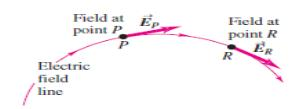QUESTION: 15

An electric field can deflect

Solution:

Only alpha rays are moving with small velocity and having charge so they will be affected by electric field.
X Rays and Gamma Rays are electromagnetic radiations. They do not carry electric charge while neutron is a charge less particle.

QUESTION: 16

For uniform electric field, field lines are:

Solution:

Uniform field lines imply that every point in space has same magnitude and direction of Electric Field. It is represented by parallel and equally spaced arrows in the direction of electric field

QUESTION: 17

An electron traveling north enters a region where the electric field is uniform and points North.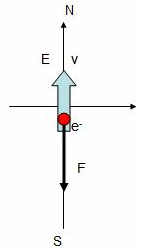Solution:

Electron is negatively charged and force is always opposite to the direction of electric field. So the when the force acts along South and opposite to the velocity which means the electron slows down.

QUESTION: 18

The magnitude of an electric field balancing a deutron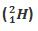of charge 1.6 x 10-19 C and mass 3.2 x 10-27 kg is:

Solution:

Given:

Charge, q = 1.6 x 10-19 C

Mass, m = 3.2 x 10-27 kg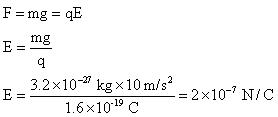QUESTION: 19

Two charges A = -2.50 μCand B = 6.0 μC are at a distance of 1 meter from each other. Distance from A at which the electric field is zero in meters is

Solution: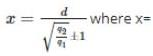distance of null point from small charge, d=distance between two charges, q2=bigger charge, q1=small charge. +1 for like charges and -1 for unlike charges x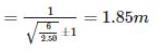QUESTION: 20

Six charges are kept at the vertices of a regular hexagon as shown in the figure. If magnitude of forceapplied by +Q on +q charge is F, then net electric force on the +Q is: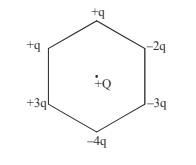Solution:

Superposition of Electrostatic Force given by Coulomb’s Law for each of the charge